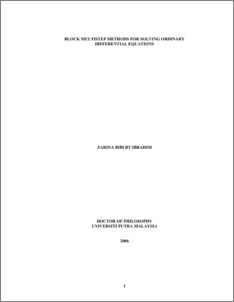# Block Multistep Methods for Solving Ordinary Differential Equations

## Citation

Ibrahim, Zarina Bibi (2006) Block Multistep Methods for Solving Ordinary Differential Equations. PhD thesis, Universiti Putra Malaysia.

## Abstract

Multistep methods for the solution of systems of Ordinary Differential Equations (ODEs) were described. The first part of the thesis is about the construction and derivation of new Block Backward Differentiation Formula (BBDF) method of constant step size and variable step size for solving first order stiff Initial Value Problems (IVPs). Their regions of stability were presented and numerical results of the methods were compared with existing methods. The second part of the thesis describes the derivation of the Adams type block method to solve second order nonstiff systems directly whilst a mixture of the Adams type formulae and the new implicit BBDF method were used to solve second order stiff problems directly. Partitioning strategies for the block method were discussed in detail and numerical results of the block partitioning are compared with the nonblock Variable Step Variable Order (VSVO) Direct Integration method for solving second order ODEs directly. Finally, this thesis deals with parallel numerical algorithms for the solution of systems of ODEs. The constructed BBDF methods are then tested and parallelism is obtained by using a Message Passing Insterface (MPI) library run on High Performance Computer (HPC). The parallel implementation of the new codes produced superlinear speedup as the dimension of the ODEs systems increased. Comparisons and illustrations with sequential codes are provided. In conclusion, the numerical results clearly demonstrates the efficiency of using the new block multistep methods for solving ODEs. Application of these multistep block method to a widely used test problems reveals the reduction in the total number of steps and execution time when compared with sequential methods.Preview
PDF
1600394.pdfView Item